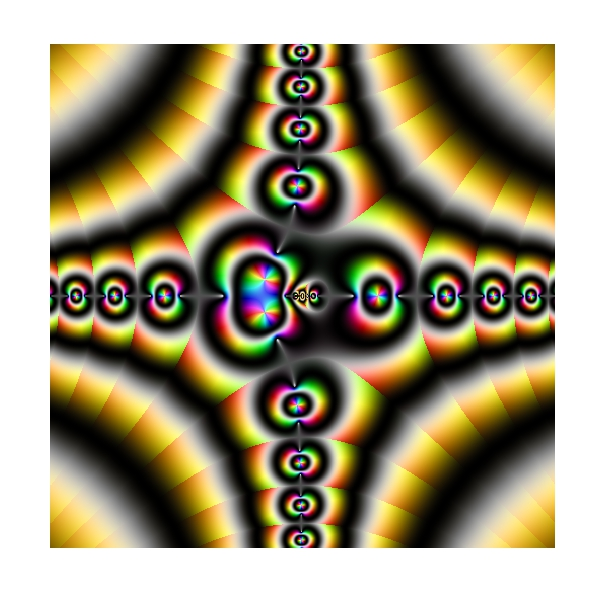# Complex Domain Coloring

Why don’t you stop doodling and start writing serious posts in your blog? (Cecilia, my beautiful wife)

Choose a function, apply it to a set of complex numbers, paint  the result using the HSV technique and be ready to be impressed because images can be absolutely amazing. You only need `ggplot2` package and your imagination. This is what happens when function is `f(x)=(1+i)log(sin((x3-1)/x))`:To learn more about complex domain coloring, you can go here. If you want to try your own functions, you can find the code below. I will try to write a serious post next time but meanwhile, long live doodles!

```require(ggplot2)
f = function(x) (1+1i)*log(sin((x^3-1)/x))
z=as.vector(outer(seq(-5, 5, by =.01),1i*seq(-5, 5, by =.01),'+'))
z=data.frame(x=Re(z),
y=Im(z),
h=(Arg(f(z))<0)*1+Arg(f(z))/(2*pi),
s=(1+sin(2*pi*log(1+Mod(f(z)))))/2,
v=(1+cos(2*pi*log(1+Mod(f(z)))))/2)
z=z[is.finite(apply(z,1,sum)),]
opt=theme(legend.position="none",
panel.background = element_blank(),
panel.grid = element_blank(),
axis.ticks=element_blank(),
axis.title=element_blank(),
axis.text =element_blank())
ggplot(data=z, aes(x=x, y=y)) + geom_tile(fill=hsv(z\$h,z\$s,z\$v))+ opt
```